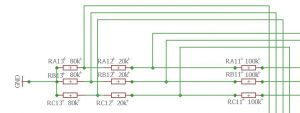Home » BLDC motor » BLDC commutation » Commutation detection at PWM high or low

## Commutation detection at PWM high or low

Jianwen Shao (thanks!) describes in this paper how the V_BEMF zero crossing detection can be done without the need to use an RC low pass filter. It can be done by just analysing the V_BEMF at times when the PWM signal ist low.
This eliminates the common mode PWM chopper signal. However at shot PWM off times at high speed of the motor, the time available to detect the zero crossing will be to low and so only maybe up to 80% PWM can be achieved. Shao then also shows that one could also detect the “zero” crossing at V_BAT / 2 on the PWM high side.

This is exactly what I am doing. So my voltage divider of the V_BEMF references at “PWM low” to GND and at “PWM high” at V_BAT / 2.I do change the voltage divider by either applying a GPIO output port that pulls Rx13 to GND (push/pull output = “0”) or redefining the same output as input and therefore do not affect the Rx12+Rx13 network.

At the same time at the reference voltage of the comparators of the STM32F303 will not be GND anymore but a voltage that corresponds to V_BAT / 2 times the voltage divider factor. Even at low speeds this method is reliable.

In SW this could look like this:

``````
void COMP1_2_3_IRQHandler(void)
{
/* USER CODE BEGIN COMP1_2_3_IRQn 0 */
if(__HAL_COMP_COMP1_EXTI_GET_FLAG() && waitForCommutation == 0)
{
HAL_COMP_Stop_IT(&hcomp1);
commutationTimerCounterArray = __HAL_TIM_GetCounter(&htim3);
commutationTimerCounterAverage = (commutationTimerCounterArray+commutationTimerCounterArray+commutationTimerCounterArray+commutationTimerCounterArray+commutationTimerCounterArray+commutationTimerCounterArray)/12; // /12
__HAL_TIM_SetCompare(&htim3,TIM_CHANNEL_1,commutationTimerCounterAverage+commutationTimerOffset);
__HAL_TIM_SetCounter(&htim3,0);
for(uint8_t i=0; i<5; i++)
{
commutationTimerCounterArray[i] = commutationTimerCounterArray[i+1];
}
waitForCommutation = 1;
}
else if(__HAL_COMP_COMP2_EXTI_GET_FLAG() && waitForCommutation == 0)
{
HAL_COMP_Stop_IT(&hcomp2);
commutationTimerCounterArray = __HAL_TIM_GetCounter(&htim3);
commutationTimerCounterAverage = (commutationTimerCounterArray+commutationTimerCounterArray+commutationTimerCounterArray+commutationTimerCounterArray+commutationTimerCounterArray+commutationTimerCounterArray)/12;
__HAL_TIM_SetCompare(&htim3,TIM_CHANNEL_1,commutationTimerCounterAverage+commutationTimerOffset);
__HAL_TIM_SetCounter(&htim3,0);
for(uint8_t i=0; i<5; i++)
{
commutationTimerCounterArray[i] = commutationTimerCounterArray[i+1];
}
waitForCommutation = 1;
}
/*
Once in this cycle we check if the speed is above or below a certain level (allow some hysteresis) to see if the comparator crossing-methode should change (pwm high or pwm low)
*/

else if(__HAL_COMP_COMP3_EXTI_GET_FLAG() && waitForCommutation == 0)
{
HAL_COMP_Stop_IT(&hcomp3);
commutationTimerCounterArray = __HAL_TIM_GetCounter(&htim3);
commutationTimerCounterAverage = (commutationTimerCounterArray+commutationTimerCounterArray+commutationTimerCounterArray+commutationTimerCounterArray+commutationTimerCounterArray+commutationTimerCounterArray)/12;
__HAL_TIM_SetCompare(&htim3,TIM_CHANNEL_1,commutationTimerCounterAverage+commutationTimerOffset);
__HAL_TIM_SetCounter(&htim3,0);
for(uint8_t i=0; i<5; i++) { commutationTimerCounterArray[i] = commutationTimerCounterArray[i+1]; } waitForCommutation = 1; if(setPWM > 300 && pwmState == 0) //300 == 50 PWM
{
GPIO_InitTypeDef GPIO_InitStruct;
GPIO_InitStruct.Pin = GPIO_PIN_5|GPIO_PIN_6|GPIO_PIN_7;
GPIO_InitStruct.Mode = GPIO_MODE_OUTPUT_PP;
GPIO_InitStruct.Pull = GPIO_NOPULL;
HAL_GPIO_Init(GPIOA, &GPIO_InitStruct);
HAL_GPIO_WritePin(GPIOA,GPIO_PIN_5,GPIO_PIN_RESET);
HAL_GPIO_WritePin(GPIOA,GPIO_PIN_6,GPIO_PIN_RESET);
HAL_GPIO_WritePin(GPIOA,GPIO_PIN_7,GPIO_PIN_RESET);

HAL_TIM_PWM_Stop(&htim1,TIM_CHANNEL_5);
HAL_DAC_SetValue(&hdac,DAC_CHANNEL_1,DAC_ALIGN_12B_R,1900+oc5Value); //4095 would be max. (3.3 V) 1241 = 1.0 V (at 6V * 20/120) or 1200 with 4S LiPo (16V)

TIM_OC_InitTypeDef sConfigOC5A;
sConfigOC5A.OCMode = TIM_OCMODE_PWM1;
compWindowOffset = -80;
sConfigOC5A.OCFastMode = TIM_OCFAST_DISABLE; //DISABLE
sConfigOC5A.OCIdleState = TIM_OCIDLESTATE_RESET;
sConfigOC5A.OCPolarity = TIM_OCPOLARITY_LOW; //LOW for PWM high detection
HAL_TIM_PWM_ConfigChannel(&htim1, &sConfigOC5A, TIM_CHANNEL_5);
TIM1->CCR5 = setPWM + compWindowOffset;
pwmState = 1;
HAL_TIM_PWM_Start(&htim1,TIM_CHANNEL_5);
}

if(setPWM > 300 && pwmState == 1 && oc5Value != oc5ValueOld)
{
HAL_DAC_SetValue(&hdac,DAC_CHANNEL_1,DAC_ALIGN_12B_R,1900+oc5Value);
oc5ValueOld = oc5Value;
}

if(setPWM <= 250 && pwmState == 1) //ca. 40 PWM
{
GPIO_InitTypeDef GPIO_InitStruct;
GPIO_InitStruct.Pin = GPIO_PIN_5|GPIO_PIN_6|GPIO_PIN_7;
GPIO_InitStruct.Mode = GPIO_MODE_INPUT;
GPIO_InitStruct.Pull = GPIO_NOPULL;
HAL_GPIO_Init(GPIOA, &GPIO_InitStruct);
HAL_TIM_PWM_Stop(&htim1,TIM_CHANNEL_5);
HAL_DAC_SetValue(&hdac,DAC_CHANNEL_1,DAC_ALIGN_12B_R,0); //4095 would be max. (3.3 V) 1241 = 1.0 V (bei 6V * 20/120)
TIM_OC_InitTypeDef sConfigOC5B;
sConfigOC5B.OCMode = TIM_OCMODE_PWM1;
compWindowOffset = 300;
sConfigOC5B.OCFastMode = TIM_OCFAST_DISABLE;
sConfigOC5B.OCIdleState = TIM_OCIDLESTATE_RESET;
sConfigOC5B.OCPolarity = TIM_OCPOLARITY_HIGH; //HIGH for PWM low detection
HAL_TIM_PWM_ConfigChannel(&htim1, &sConfigOC5B, TIM_CHANNEL_5);
TIM1->CCR5 = setPWM + compWindowOffset;
pwmState = 0;
HAL_TIM_PWM_Start(&htim1,TIM_CHANNEL_5);
}
}
``````

The above function is the interrupt routine of the comparator interrupt.

The STM32F303 allows to set a comparator window to make him blind during a certain time. I use this to blank out the switching phase and to allow for the transients to settle. This window derives from the PWM counter and is different at PWM low or PWM high.

The reference voltage for the comparator is set with a DAC channel. PWM low about GND, PWM high at about 1,8 V.

Right after the interrupt routine detected a comparator state change, I do perform the switch over (with hysteresis) between PWM low and PWM high because that gives me some time until I have to be ready for the next commutation detection.

Between the zero-crossing and the commutation of the motors a 30º delay has to be implemented. I do measure with an other counter the time between the commutations. This counter value / 2 is then used to fire with another interrupt the motor commutation.

I do also average this time measurement for smoother loop performance.